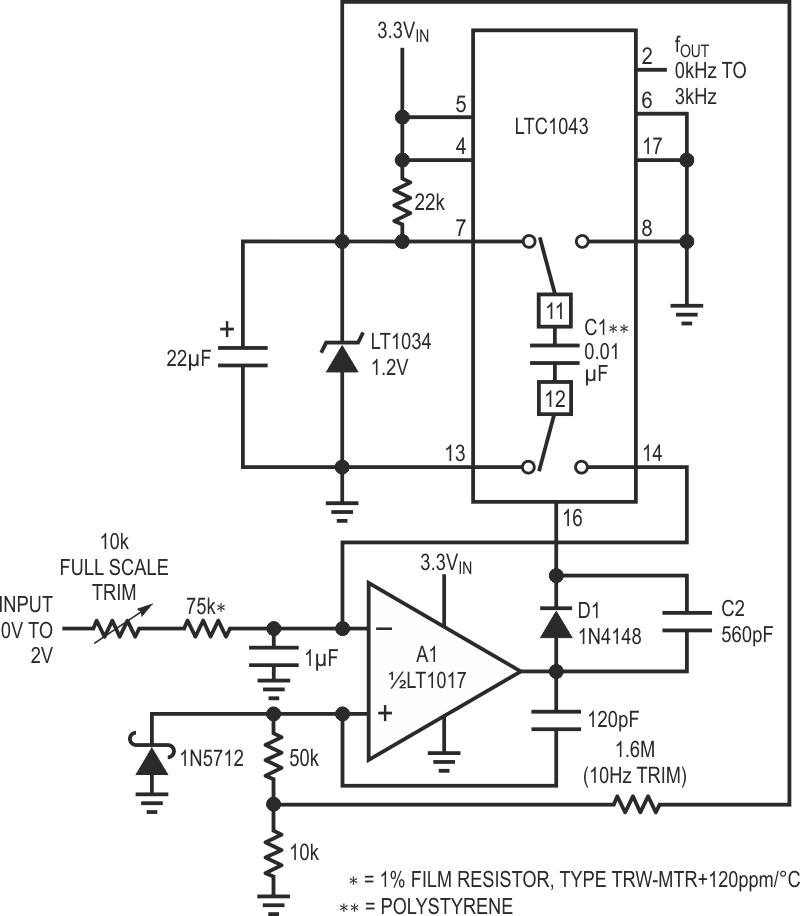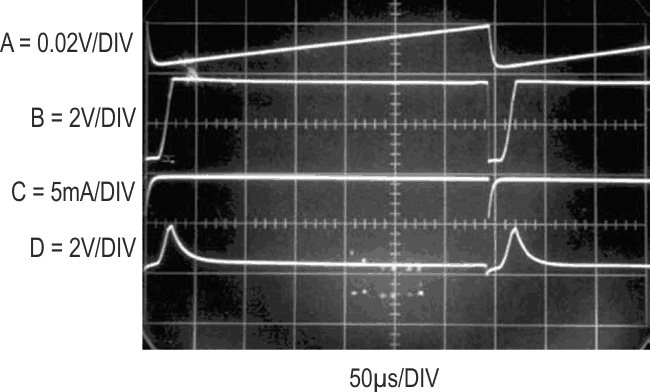# 3.3V powered V/F converter

## Analog Devices LT1017 LT1034 LTC1043

Figure 1 is a “charge pump” type V/F converter specifically designed to run from a 3.3 V rail. A 0 V to 2 V input produces a corresponding 0 kHz to 3 kHz output with linearity inside 0.05%. To understand how the circuit works assume that A1’s negative input is just below 0 V. The amplifier output is positive. Under these conditions, LTC1043’s pins 12 and 13 are shorted as are pins 11 and 7, allowing the 0.01 μF capacitor (C1) to charge to the 1.2 V LT1034 reference. When the input-voltage-derived current ramps A1’s summing point (negative input-trace A, Figure 2) positive, its output (trace B) goes low. This reverses the LTC1043’s switch states, connecting pins 12 and 14, and 11 and 8. This effectively connects C1’s positively charged end to ground on pin 8, forcing current to flow from A1’s summing junction into C1 via LTC1043 pin 14 (pin 14’s current is trace C). This action resets A1’s summing point to a small negative potential (again, trace A). The 120 pF-50k-10k time constant at A1’s positive input ensures A1 remains low long enough for C1 to completely discharge (A1’s positive input is trace D). The Schottky diode prevents excessive negative excursions due to the 120 pF capacitors differentiated response.

When the 120 pF positive feedback path decays, A1’s output returns positive and the entire cycle repeats. The oscillation frequency of this action is directly related to the input voltage.Figure 1. 3.3 V powered voltage-to-frequency converter. Charge pump based feedback maintains high linearity and stability.

This is an AC coupled feedback loop. Because of this, start-up or overdrive conditions could force A1 to go low and stay there. When A1’s output is low the LTC1043’s internal oscillator sees C2 and will begin oscillation if A1 remains low long enough. This oscillation causes charge pumping action via the LTC1043-C1-A1 summing junction path until normal operation commences. During normal operation A1 is never low long enough for oscillation to occur, and controls the LTC1043 switch states via D1.Figure 2. Waveform for the 3.3 V powered V/F. Charge pump action (Trace C) maintains summing point (Trace A), enforcing high linearity and accuracy.

To calibrate this circuit apply 7 mV and select the 1.6M (nominal) value for 10 Hz out. Then apply 2.000 V and set the 10k trim for exactly 3 kHz output. Pertinent specifications include linearity of 0.05%, power supply rejection of 0.04 %/V, temperature coefficient of 75 ppm/°C of scale and supply current of about 200 μA. The power supply may vary from 2.6 V to 4.0 V with no degradation of these specifications. If degraded temperature coefficients are acceptable, the film resistor specified may be replaced by a standard 1% film resistor. The type called out has a temperature characteristic that opposes C1’s –120 ppm/°C drift, resulting in the low overall circuit drift noted.